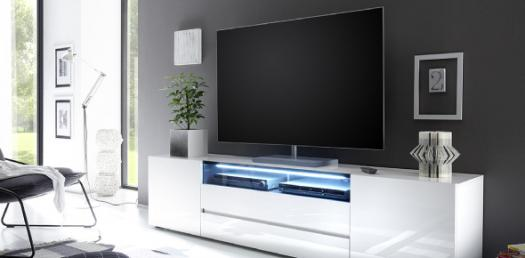# Grob TV - Basic Television Multiple Choice

28 Questions | Total Attempts: 385SettingsRelated Topics
• 1.
Which of the following camera tube uses lead oxide (PbO) for the photoconductive target plate?
• A.

Vidicon

• B.

Plumbicon

• C.

Saticon

• D.

Image Orthicon

• 2.
Camera signal output without sync is called
• A.

Black burst

• B.

Generator lock video

• C.

Composite video

• D.

Noncomposite video

• 3.
• A.

Low beam current

• B.

High gain in the amplifier

• C.

Excessive gamma

• D.

Insufficient scanning width

• 4.
Which of the following camera tubes has minimum lag?
• A.

Vidicon

• B.

Plumbicon

• C.

Saticon

• D.

Iconoscope

• 5.
• A.

Red

• B.

Blue

• C.

Yellow-green

• D.

Infrared

• 6.
Precise scanning size and linearity are most important in
• A.

A black-and-white camera

• B.

A plumbicon

• C.

A single-tube color pickup

• D.

A saticon

• 7.
Beam alignment magnets for the camera tube are adjusted while rocking which control?
• A.

Optical focus

• B.

Electrical focus

• C.

Beam current

• D.

• 8.
• A.

CCU

• B.

ENG camera

• C.

SEG

• D.

Sync generator

• 9.
• A.

0.4545

• B.

1.0

• C.

1.4

• D.

2.2

• 10.
If the camera cannot be placed far away enough to include everything in the scene, change the lens to one with a
• A.

Lower f rating

• B.

Higher f rating

• C.

Longer focal length

• D.

Shorter focal length

• 11.
A typically value of vidicon dark current is
• A.

0.2 uA

• B.

• C.

8 mA

• D.

800 mA

• 12.
• A.

2

• B.

4

• C.

8

• D.

32

• 13.
Which of the following is FALSE?
• A.

The lens inverts the optical image on the faceplate of the camera tube.

• B.

The composite video signal includes the camera signal and sync not blanking

• C.

The standard composite video from a camera is 1V p-p with negative sync.

• D.

The plumbicon uses a lead oxide layer for the target plate.

• 14.
Which of the following is TRUE?
• A.

The diameter of the vidicon image plate is about 5 in. (127 mm)

• B.

The plumbicon camera tube uses a silicon target plate.

• C.

The composite video signal includes the camera signal and sync but not blanking.

• D.

The lens inverts the optical image on the faceplates of the camera tube.

• 15.
Which of the following produces the signal variations for G4 of the vidicon.
• A.

Target plate

• B.

Wire mesh

• C.

Muzzle

• D.

Beam control

• 16.
What is the gamma required for the camera tube?
• A.

2.2

• B.

0.4545

• C.

4.545

• D.

0.22

• 17.
is more important for the gamma correction for the camera tube.
• A.

Color

• B.

Monochrome

• C.

Both Color and monochrome

• D.

Either of Color or monochrome

• 18.
In gamma correction the             is stretched by the picture tube.
• A.

Black

• B.

Gray

• C.

White

• D.

Red

• 19.
To how many lax units is the illuminator of 3 fs, approximately equal?
• A.

650

• B.

225

• C.

65

• D.

30

• 20.
What is the diagonal screen size for the 19CP4 picture tube?
• A.

12 in.

• B.

16 in.

• C.

19 in.

• D.

24 in.

• 21.
To what deflection angle does a maximum deflection angle of 45 deg either side center correspond?
• A.

30º

• B.

90º

• C.

120º

• D.

360º

• 22.
What is the usual heater voltage for picture tube?
• A.

1.6 v

• B.

5.6 V

• C.

6.3 V

• D.

9.3V

• 23.
What is the typical anode voltage for a 25-in color picture tube?
• A.

10 kV

• B.

30 kV

• C.

1 kV

• D.

30 V

• 24.
Typically, the anode capacitance for a 25-in tube is
• A.

2

• B.

20

• C.

205

• D.

2000

• 25.
When the TV set was turned on, full power was applied to the heater and the picture appeared within a fraction of a second.
• A.

Ultor

• B.

Implosion

• C.

Instant-on operation

• D.

Screen persistence

• 26.
What are the phosphor numbers, respectively, for monochrome and color picture tubes?
• A.

P1 and P4

• B.

P1 and P22

• C.

P4 and P22

• D.

P4 and P1

• 27.
What is the color of P1 screen phosphor?
• A.

Red

• B.

Blue

• C.

White

• D.

Green

• 28.
Which of the following is the most negative (or least positive) electrode is the electron gun?
• A.

Cathode

• B.

Control grid G1

• C.

Screen grid

• D.

Focus grid